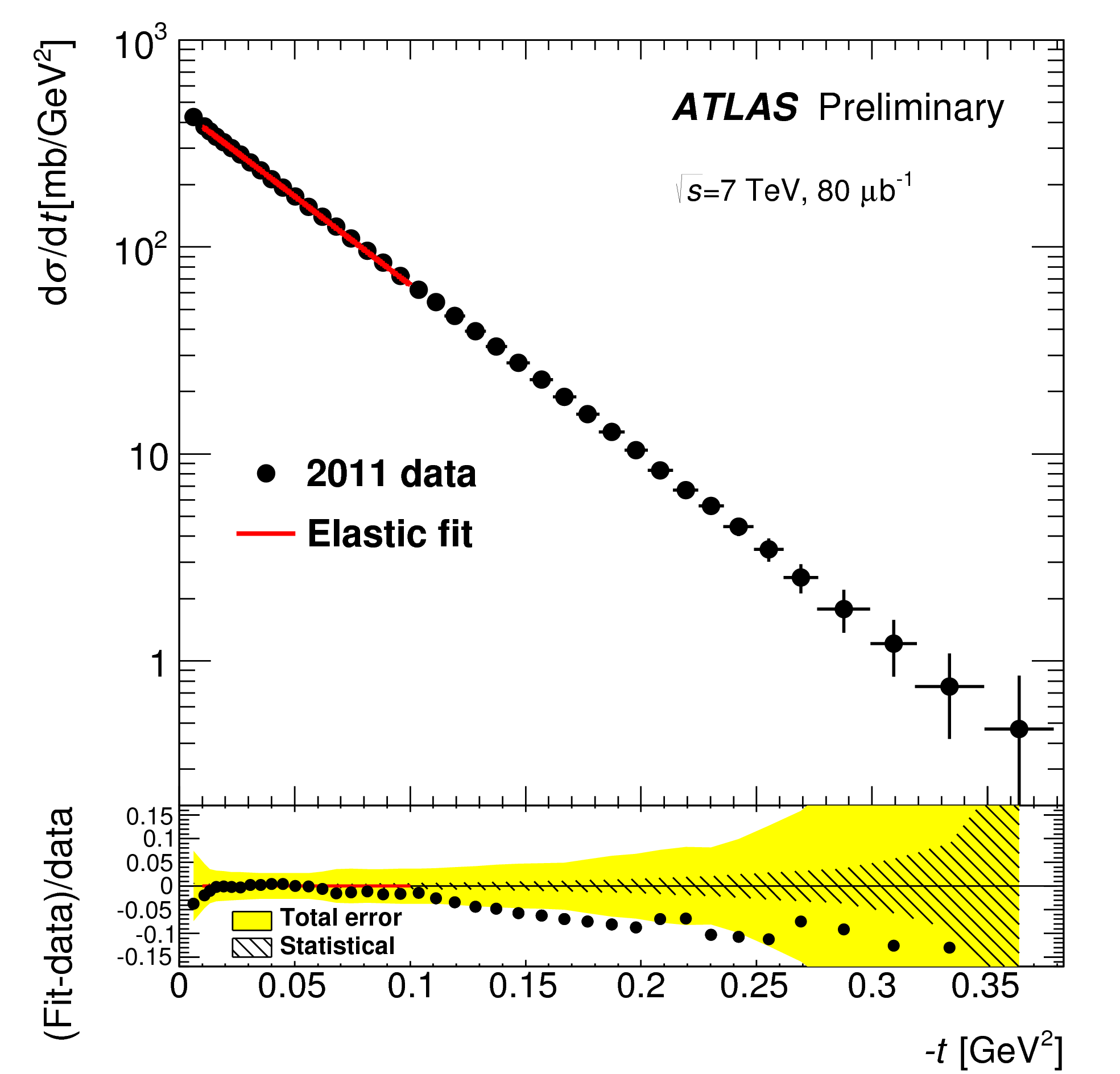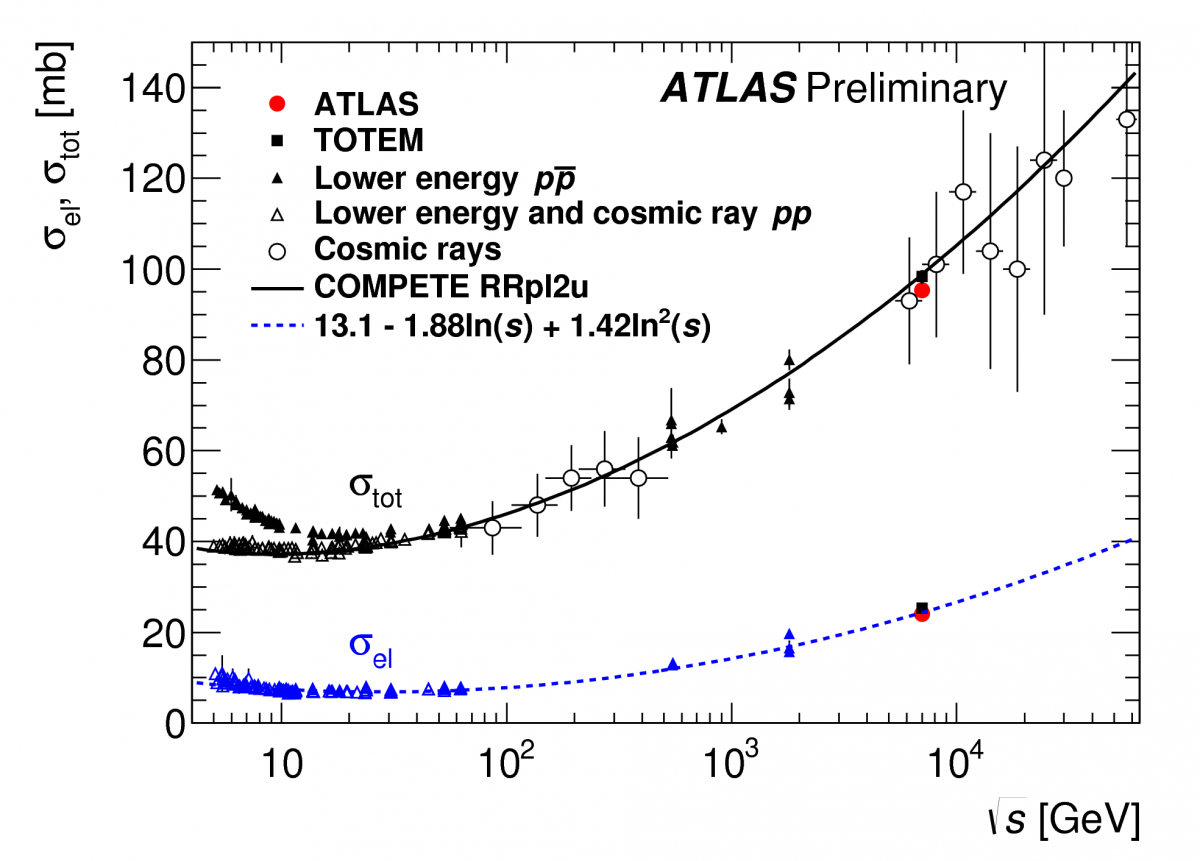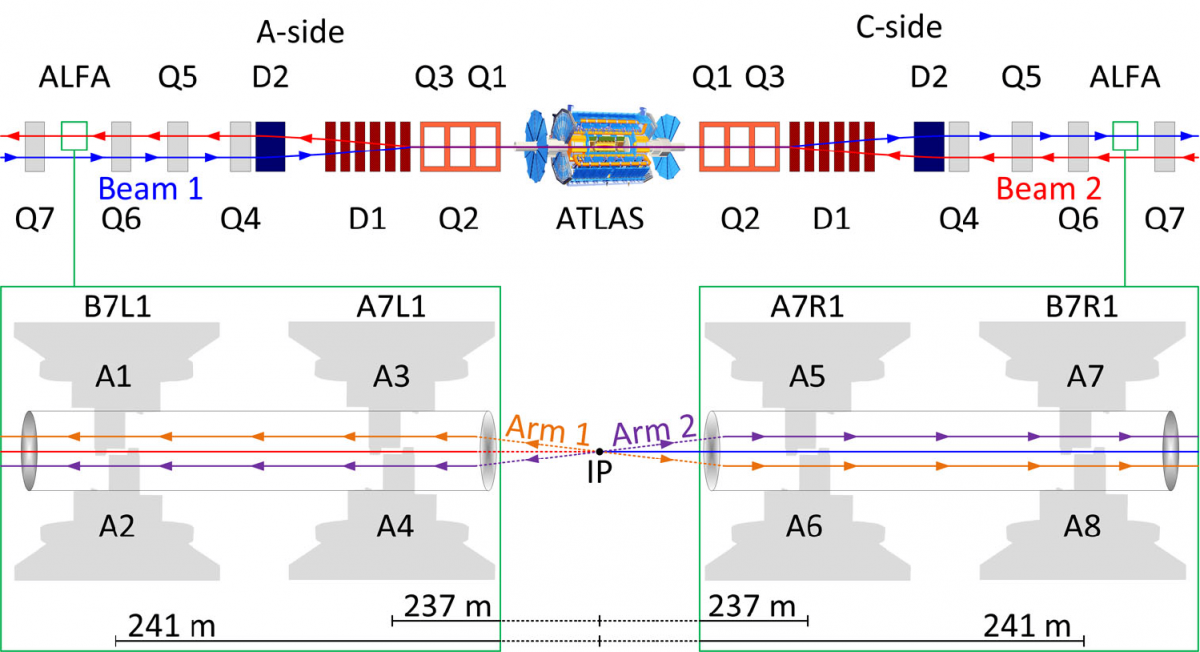The measured differential elastic cross section and the fit used to extract the total cross section. (Image: ATLAS Experiment/CERN)The ATLAS measurement of the total and elastic cross sections compared to other measurements and model predictions. (Image: ATLAS Experiment/CERN)

Data from a special run of the LHC using dedicated beam optics at 7 TeV have been analysed to measure the total cross-section of proton-proton collisions in ATLAS. Using the Absolute Luminosity For ATLAS (ALFA), a Roman Pot sub-detector located 240 metres from the collision point, ATLAS has determined the cross-section with unprecedented precision to be σtot (pp → X) = 95.4 ± 1.4 millibarn.

The total cross-section is a fundamental parameter of the strong interactions, setting the scale of the size of the interaction region at a given energy. To measure the total cross-section, we employ the optical theorem, which states that the total cross-section is proportional to the imaginary part of the forward elastic scattering amplitude, extrapolated to t = 0. That is, if we measure the elastic scattering cross-section differential in the momentum-transfer variable t, then we can calculate the total cross-section.A sketch of the experimental set-up, not to scale, showing the positions of the ALFA Roman Pot stations in the outgoing LHC beams, and the magnetic elements situated between the interaction point and ALFA. (Image: ATLAS Experiment/CERN)

Measuring the elastic scattering is a challenge because elastically-scattered protons (protons that essentially bounce off of each other) escape the interaction under very small angles of tens of micro-radians. To detect these protons, dedicated detectors are installed, such as ALFA. To achieve the required focusing properties, the LHC must be operated with special settings of its magnets. The detectors can then be moved as close as a few millimeters from the LHC beam to access the smallest scattering angles.